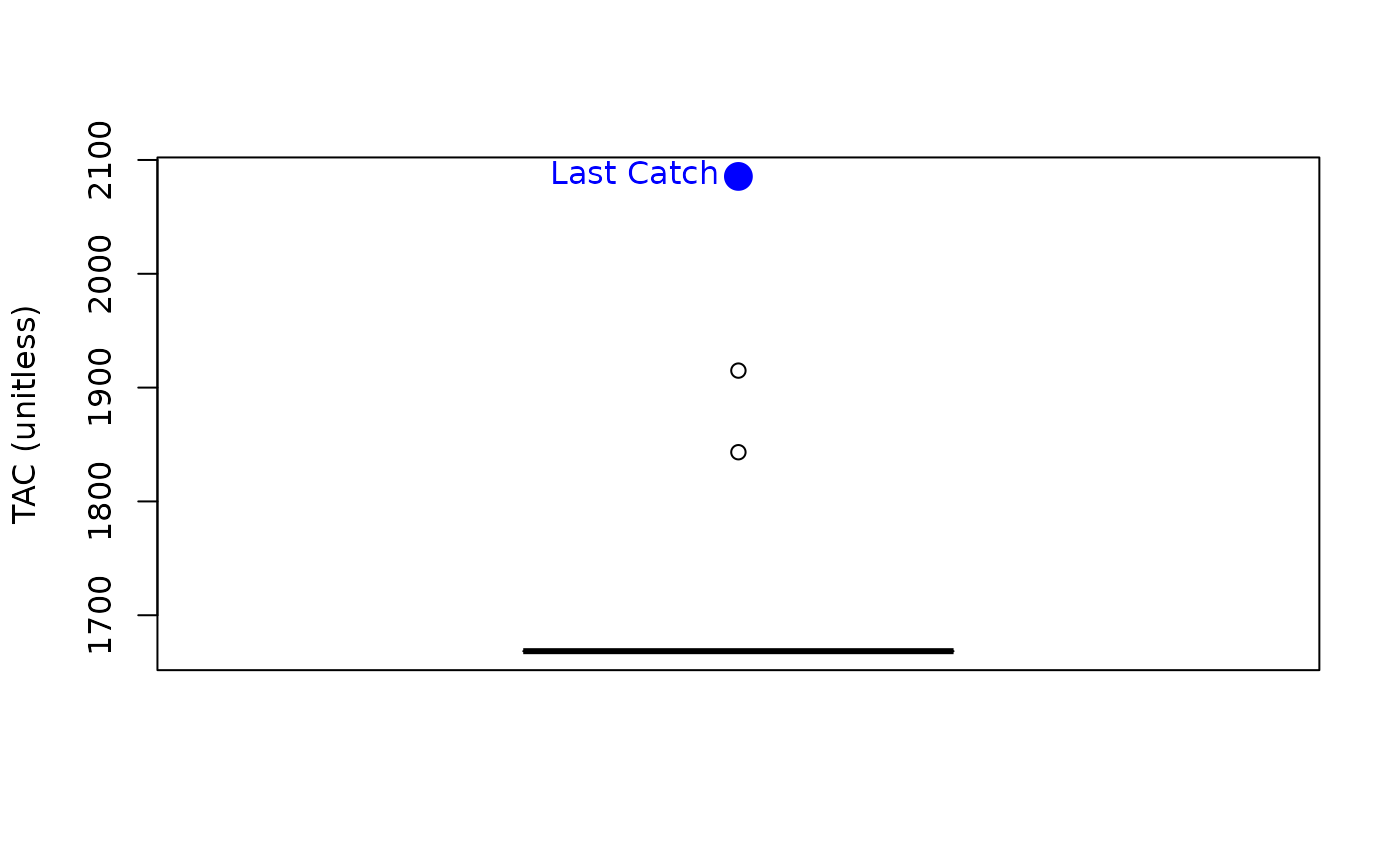A simple MP that aims for a reference catch (as a proxy for MSY) subject to imperfect information.

GB_CC(x, Data, reps = 100, plot = FALSE)

## Arguments

x

A position in the data object

Data

A data object

reps

The number of stochastic samples of the MP recommendation(s)

plot

Logical. Show the plot?

## Value

An object of class Rec-class with the TAC slot populated with a numeric vector of length reps

## Details

Note that this is my interpretation of their MP and is now stochastic. Currently it is generalized and is not 'tuned' to more detailed assessment data which might explain why in some cases it leads to stock declines.

The TAC is calculated as: $$\textrm{TAC} = C_\textrm{ref}$$ where $$C_\textrm{ref}$$ is a reference catch assumed to be a proxy for MSY. In the MSE $$C_\textrm{ref}$$ is the calculated MSY subject to observation error defined in Obs@CV_Cref.

The TAC is subject to the following conditions:

1. if next TAC > 1.2 last catch, then TAC = 1.2 last catch

2. if next TAC < 0.8 last catch, then TAC = 0.8 last catch

## Required Data

See Data-class for information on the Data object

GB_CC: Cref

## Rendered Equations

See Online Documentation for correctly rendered equations

Other Constant Catch MPs: CC1()

T. Carruthers

## Examples

GB_CC(1, MSEtool::SimulatedData, plot=TRUE)#> TAC (median)
#>     1668.429